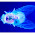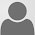Wednesday, June 6, 2012

Body Weight in the United States - Part 1, "The Problem"

The Problem

In the United States, people are getting fatter and they are doing so at an alarming rate.

So What?

Being fat isn't just a social stigma - it's unhealthy in nearly every facet of life. Staying in this condition brings high blood pressure, diabetes, asthma and arthritis to name but a few. Additionally, an obese person has a much harder time 'doing stuff' in terms of physical activity.

Want to know what it feels like? Strap on a fifty pound backpack and go for a walk. Better yet, try running with that extra weight and you will see just how much it impacts your ability to exercise let alone your ability to enjoy it. Do this daily for years and your joints will be set up perfectly for a lifetime of pain and stiffness.

Kudos to our military personnel (and any other job where this is required) who wear at least that much gear every day while performing their duties.

For the rest of us, an honest assessment is needed to reverse this trend.

BMI

Do you know your BMI (Body Mass Index)? Click here to access the National Heart, Lung and Blood Institute's calculator.

Once you have your BMI, look at the above chart. Where do you stand? If you are muscular, the calculator tends to overestimate while if you are older it may underestimate. That being said, it will still be in the ballpark because humans all share the same basic anatomy.

Heavier People

Ignoring BMI and only looking at body weight, it is obvious that people are heavier. Adult men on average have gained about 25 lbs (11.3 kg) from 1960 through 2002:Average weight for adult men.

That's about 0.6 lbs (0.27 kg) per year. Like many trends, it doesn't seem so bad when describing the rate, but over time it adds up rather quickly.

Taller People

Since 1960, people have grown taller - about 0.9 inches (2.29 cm) taller for women and about 1.1 inches (2.79 cm) taller for men. So we are taller and heavier. That would explain some weight gain, but does it account for all 25 lbs? A little bit of math will reveal the answer:

1960:                    166.3 lbs / 68.3 inches    2.43 lbs/inch

2002:                    191.0 lbs / 69.4 inches    2.75 lbs/inch

The above calculations show that we are heavier per inch of height - about 1/3 lb more. Therefore the extra height does not explain all of the extra weight gain.

How much should an average height male weigh today?

If we were to measure how much we would weigh in 1960 given our extra height, it would come out to about 169 lbs! Compare that to 191 lbs - a difference of 22 lbs (10 Kg)!

Conclusion

People in the United States are getting taller and heavier. The rate of the former (height) is much slower than the latter (weight). People are becoming more unhealthy as a result. Additionally, it can be difficult to enjoy life when toting around those extra pounds. The only resolution to this problem is to lose weight - permanently. The next two articles will present information why this trend has occurred while also shedding light on ways to possibly reverse it.

Questions:
1) How long can this trend continue?
2) What is the burden on health care due to obesity?
3) Are other countries experiencing similar problems?

Data:
Code:
These graphs were generated using the 'ggplot2' and 'maps' packages within the R programming language. Additional graphics were created/edited using GIMP.

1st graph:
ggplot() +
geom_line(data = subset(bmi.frame, Description == "Obese (BMI >= 30.0)"), aes(x=Year, y=Median, color="Obese (BMI >= 30.0)"), size=2) +
geom_line(data = subset(bmi.frame, Description == "Overweight (BMI >= 25.0 and BMI <= 29.9)"), aes(x=Year, y=Median, color="Overweight (BMI >= 25.0 and BMI <= 29.9)"), size=1) +
geom_line(data = subset(bmi.frame, Description == "Neither overweight nor obese (BMI <= 24.9)"), aes(x=Year, y=Median, color="Neither overweight nor obese (BMI <= 24.9)"), size=1) +

geom_point(data = subset(bmi.frame, Description == "Obese (BMI >= 30.0)"), aes(x=Year, y=Median, color="Obese (BMI >= 30.0)"), size=3) +
geom_point(data = subset(bmi.frame, Description == "Overweight (BMI >= 25.0 and BMI <= 29.9)"), aes(x=Year, y=Median, color="Overweight (BMI >= 25.0 and BMI <= 29.9)"), size=2) +
geom_point(data = subset(bmi.frame, Description == "Neither overweight nor obese (BMI <= 24.9)"), aes(x=Year, y=Median, color="Neither overweight nor obese (BMI <= 24.9)"), size=2) +

ylab("% of Population") +
xlab("Year") +

scale_colour_manual(values=cbPaletteNoGray) +

opts(title="Body Mass Index (BMI) in the United States",
legend.title = theme_blank(),
panel.background = theme_blank(),
axis.line = theme_segment())

2nd graph:
ggplot(weight.frame, aes(x=Date.Range, y=Weight.lb)) +
geom_point(aes(size=Weight.lb), color="dark red") +
geom_line(aes(group=1, size=Weight.lb), color="dark red") +
geom_smooth(aes(group=1), size=1, color="black", method="lm", na.rm=FALSE, fill=NA, linetype=2) +

ylab("Weight (pounds)") +
xlab("Year Range") +

opts(title="Weight Gain in the United States",
legend.title = theme_blank(),
panel.background = theme_blank(),
axis.line = theme_segment())

1.I think your calculation of weight per height is not correct. There is a allometric effect you should take in account: Doubled height means squared volumina - therefore weight-gain must be more than linear.

1.I'm not sure the allometric effect works here since we're talking about the same species (humans). Using your argument, if a newborn baby is 18 inches tall and weighs 7 lbs (average figures), then by the time they are 36 inches tall (3 feet), they should weight 49 lbs - okay there.

BUT - assuming they reach 72 inches (6 feet), by your reasoning they should weight 343 lbs!

So, while there might be a slight allometric effect in play, it certainly isn't enough to worry about here and will not make up the 22 lb difference mentioned in the article.

2.yeah - you're right... thanks for the reply!

2.There _is_ an allometric effect, and your linear fit would be dismissed by anyone who deals with obesity in prevalence studies.

By Patrick's reasoning (linear relationship), either:
18" 7 lbs
double: 36" = 14 lbs
quadruple: 72" = 28 lbs
or, if you keep your averages from adults, your 2.75 lb/" would have that 18" newborn weigh 49.5 lbs (ouch!).

If the shape was similar (a cube for simplicity, or a baby scaled up to a pudgy adult), the volume (and thus mass) would scale with the cube (^3) of a length: doubling the height would mean 8x the weight.
18" to 36" = 2x so weight would be 7 * 8 = 56
18" to 72" = 4x so 7 * 64 = 448, even greater than 343
But teenage let alone adult humans are not pudgy shaped like infants: as humans grow, they become more elongated, so the empirical exponent is less than 3 (the empirical exponent differs among species).

For adults humans, the standard correction to make a scalar obesity index (Quetelet, often BMI) is weight / height^2 (using kg and cm, or multiplied by 703 if you use lbs & inches); the exponent of 2 is pretty close to the empirical exponent. The major advantage of using Quetelets for your comparison is that they do a better job of capturing the variation among people at a given time: the BMI calculated form the average weight and average height will not be the same as the average of BMIs calculated on each individual.

If anyone finds this post while searching for obesity, I encourage you to look at how the field deals with scaling weight for height.

On a graph style note, given that your Y axis are percentages that add to 100, I think that your first graph would be less misleading if the Y axis ran from 0 to 50. The way you scaled it, the visual impression is that in 1995, the prevalence of obese is roughly 1/10th of overweight.

1.Good info.

Regarding the first graph, I didn't 'scale' anything. I cropped the area to show the relevant data - completely different than scaling. I try very hard *not* to produce misleading graphs - there are enough of those in mainstream media. :-)